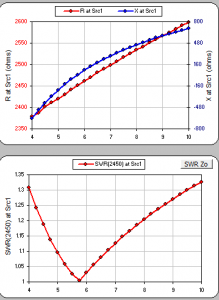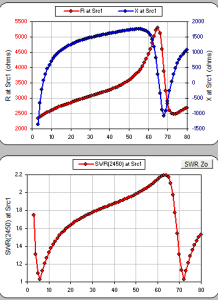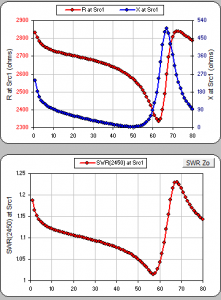# 160meter EndFed HalfWave Antenna on a 65ft tower

Discussion in 'Antennas, Feedlines, Towers & Rotors' started by WA7ARK, Jun 13, 2019.

Tags:1. Yes, the wire is 5.71ft+64.78ft = 70.49ft long. At 7.15Mhz, wl=137.56ft, therefore 70.49/137.56 = 0.512 lambda.

Not 10.23%. The distance from the feedpoint to the short end is 5.71ft. 5.71/70.49 = 0.081 = 8.1% from the end.
How are you placing the source. Remember that it has to be at the center of a segment. This is not necessarily some arbitrary percentage along a conductor. Did you use my segmentation or did you create your own?
Again, here are the details of the three wires I am using to represent the antenna. The source is in the center of wire W2:You cannot model this as a single wire. If you are doing that, then your results will not match mine, besides wire #1 is the diameter of the coax it is made of.

Ditto, except that I defined the antenna as lying along the Y-axis, not the X-axis. Which means you are not using my wires.

2. I didn't change your geometry, segments, source placement, or any other file definitions in any way.

The radiation pattern is 3-dimensional, and will have a response along the x AND the y axes. I gave the gain along the x axis because it is the peak gain from that physical orientation of that antenna.

The edit I made to my post about your file shows the source placed 8.1% from the nearer end.

Last edited: Aug 26, 2019
3. Ok, I accounted for the difference in the pattern. I run EzNec in the mode where azimuths are plotted using compass mode. In any case, the maximum radiation is broadside to the wire, and Nec2 and Nec4 come up with identical broadside gains...

On the matter of the slightly different values of feed point impedance.
Nec2 > 2450 - j2.69 = 2450@0.07derg
Nec4 > 2440 +j92.3 = 2441.7@2deg

That means that the magnitudes of the impedance are within 8.3/2442 = 0.3%, which means that the two algorithms are very close to each other for free space impedance calculations.

4. Now that we have established that a 70.49ft long #14 wire fed 5.71 ft from one end presents a free-space impedance close to 2450+j0 Ohms at 7.150MHz, I think it is interesting to show what happens if everything stays the same while we change only the length of the short (5.71ft) wire. Remember that the short wire represents the outside of the coax that normally feeds this antenna. This is to show that the swr of the this antenna is not very sensitive to this length.

LEFT plots, I vary the short wire from 4 to 10ft in steps of 0.25ft (3inches).
RIGHT plots, I vary the short wire from 5 to 70ft in steps of 1ft. (click to enlarge these plots)The takeaways:
Short wire = (5.7ft +- a couple of feet) changes the Swr hardly at all.
As the short wire's length is changed through a half wavelength, the worst Swr peaks at 2.2. The R,jX, and Swr are periodic, and repeat for each half-wavelength of added coax length. This means that if you just add random length of coax (the short wire), the Swr will never be more than 2.2 and it could be a lot less.

My premise that it is a good idea to put a Common-Mode-Choke a short distance down the coax from the feedpoint. Using values for R and X from G3TXQ's choke data page, I added a choke onto the coax. First, I optimized the choke placement when the coax feed-line is 50ft long (where the un-choked Swr2450 was ~2). The choke position that minimizes Swr2450 is 7.5ft from the transformer. Now, let's see what happens to the Swr as the length of coax beyond the choke is changed. I step the length of the coax (from the new Choke to the end) from 1ft to 80ft in steps of 1ft:Notice how adding the Choke 7.5ft from the feedpoint has stabilized the Swr plot (Swr is now always less than 1.2). This explains why adding the Choke worked so well in the 160m antenna presented in post #1 of this thread.